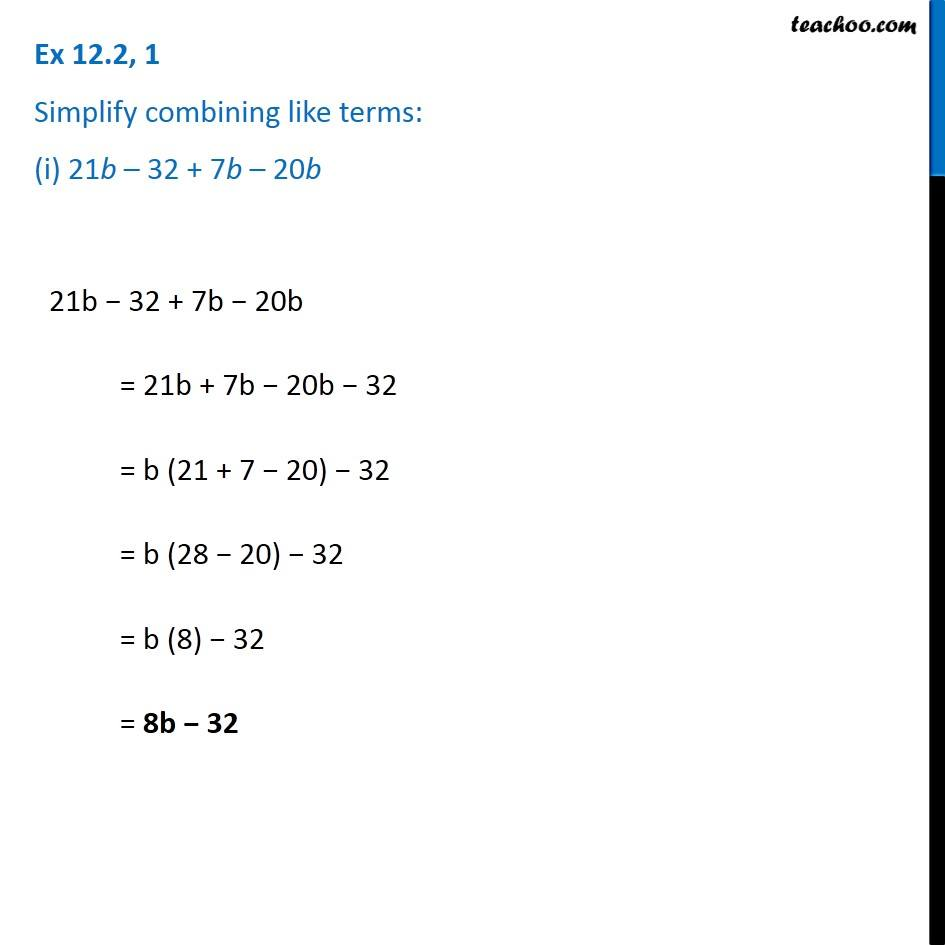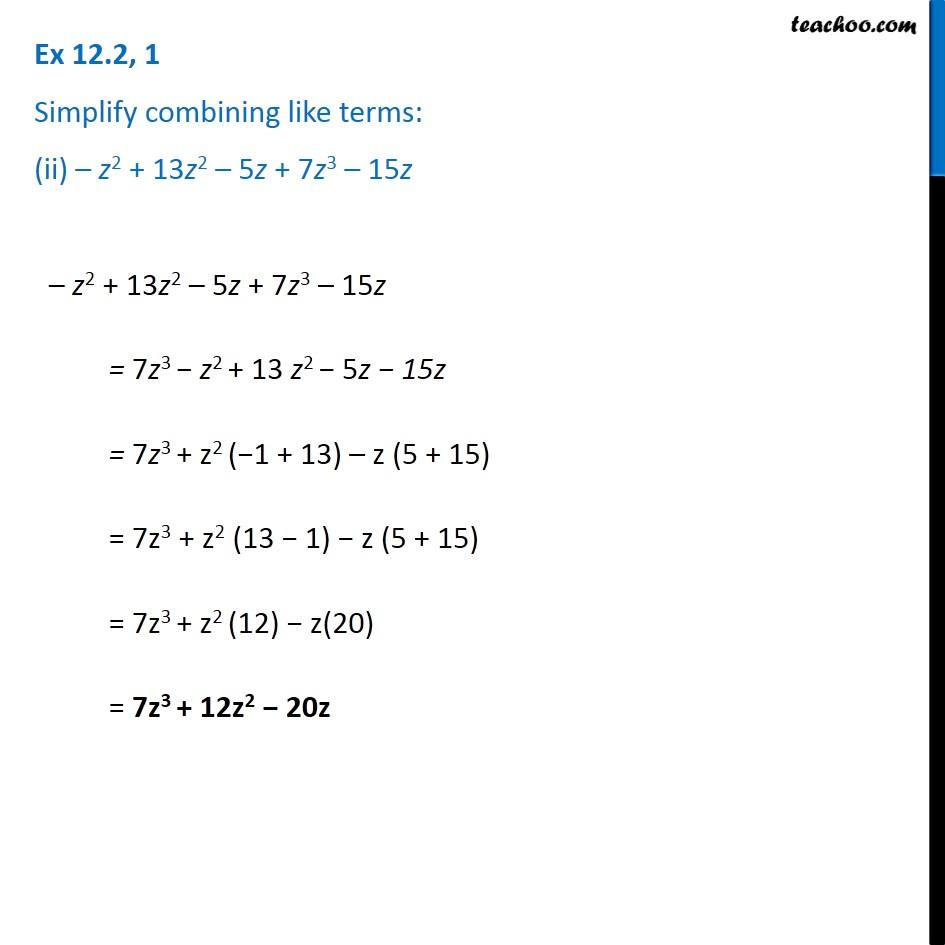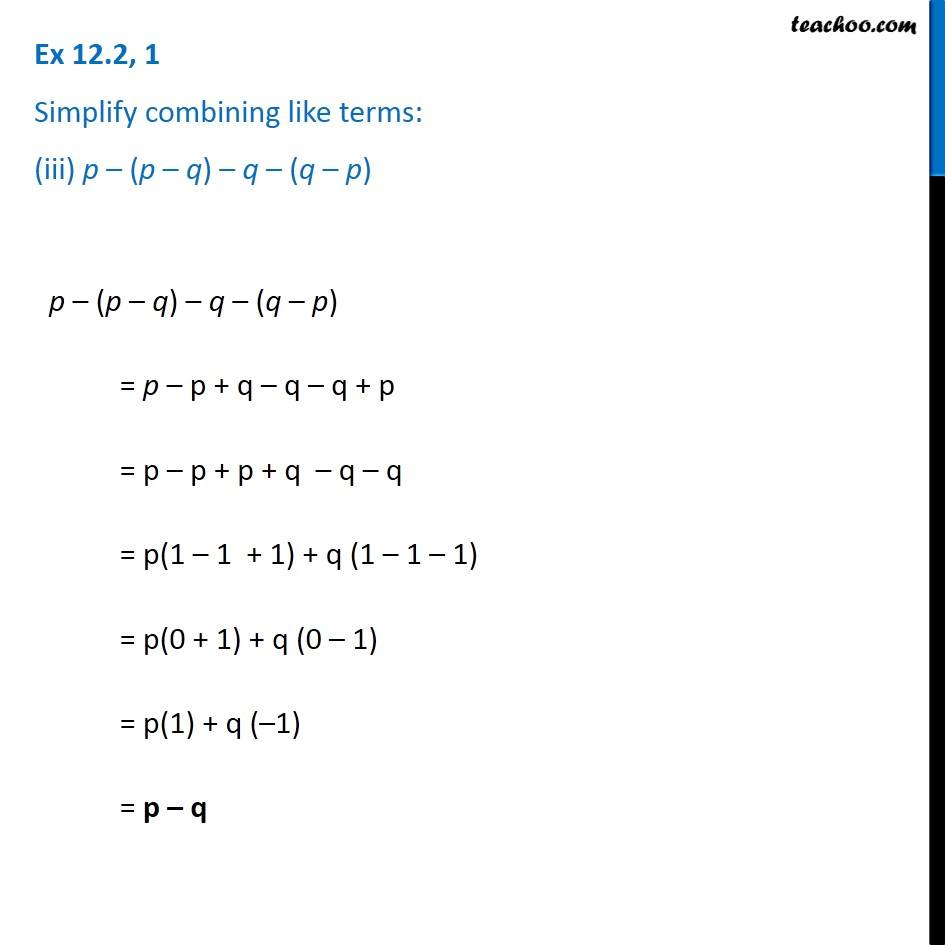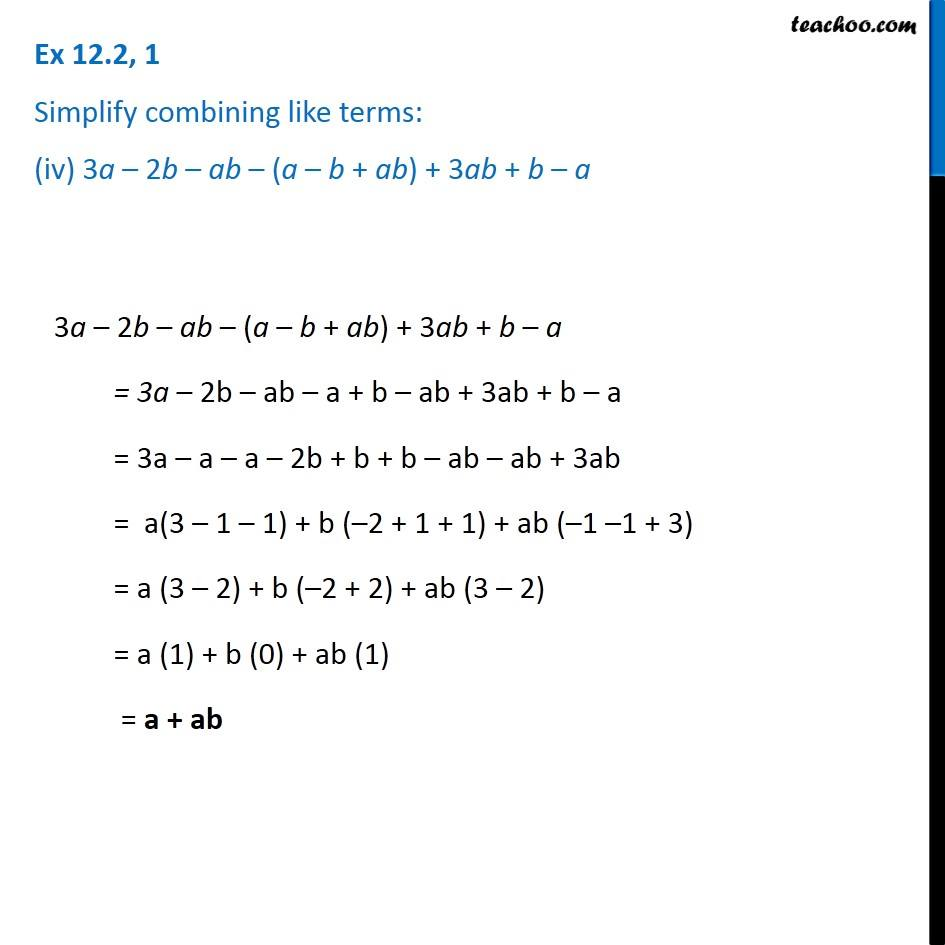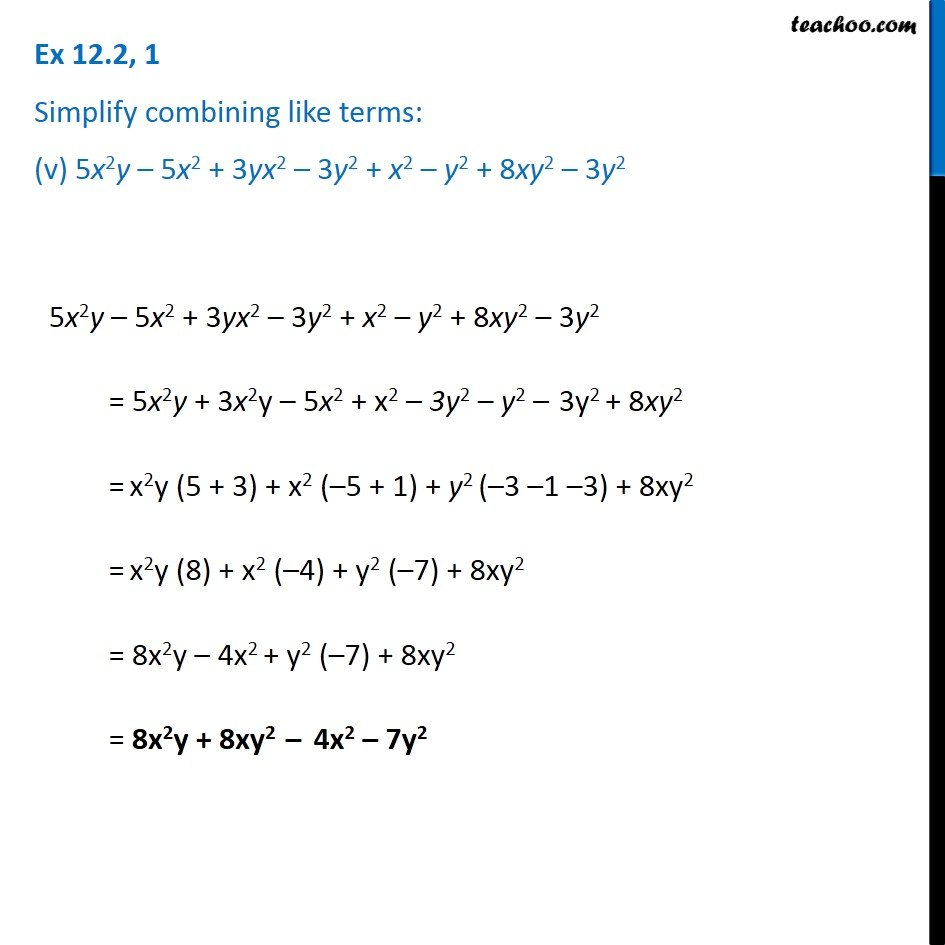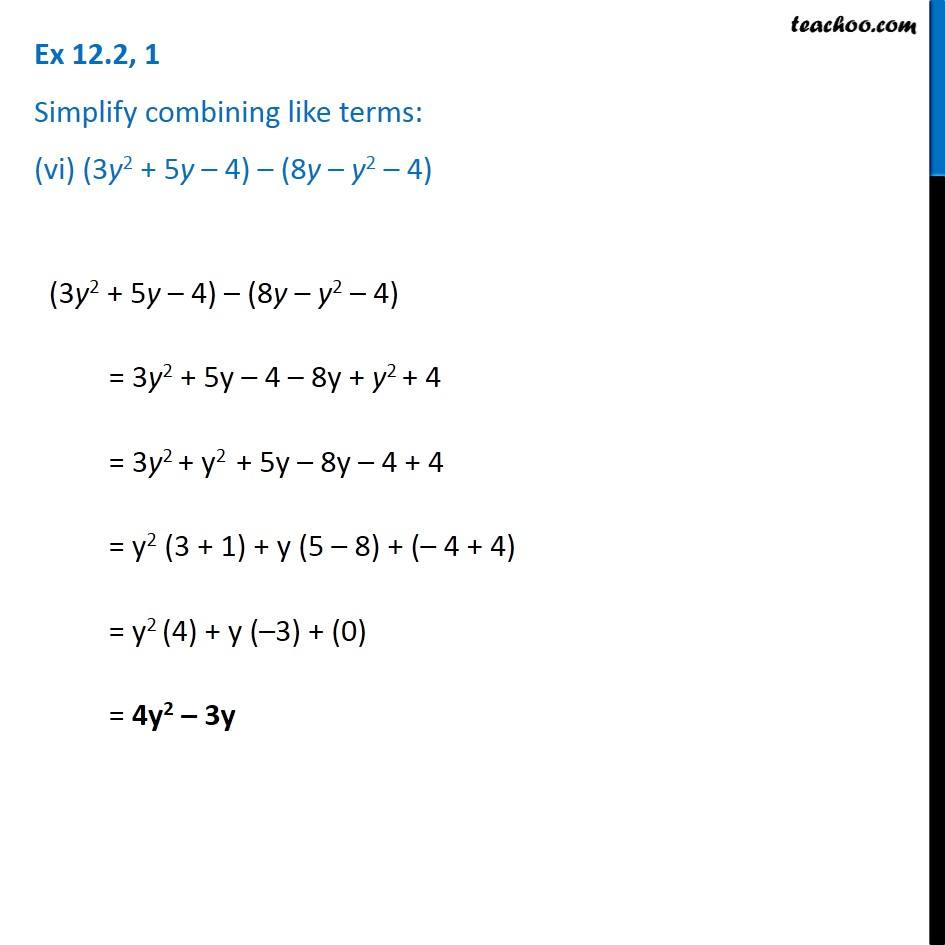1. Chapter 12 Class 7 Algebraic Expressions
2. Concept wise
3. Addition and Subtraction of Algebraic Expressions

Transcript

Ex 12.2, 1 Simplify combining like terms: (i) 21b – 32 + 7b – 20b 21b − 32 + 7b − 20b = 21b + 7b − 20b − 32 = b (21 + 7 − 20) − 32 = b (28 − 20) − 32 = b (8) − 32 = 8b − 32 Ex 12.2, 1 Simplify combining like terms: (ii) – z2 + 13z2 – 5z + 7z3 – 15z – z2 + 13z2 – 5z + 7z3 – 15z = 7z3 − z2 + 13 z2 − 5z − 15z = 7z3 + z2 (−1 + 13) – z (5 + 15) = 7z3 + z2 (13 − 1) − z (5 + 15) = 7z3 + z2 (12) − z(20) = 7z3 + 12z2 − 20z Ex 12.2, 1 Simplify combining like terms: (iii) p – (p – q) – q – (q – p) p – (p – q) – q – (q – p) = p – p + q – q – q + p = p – p + p + q – q – q = p(1 – 1 + 1) + q (1 – 1 – 1) = p(0 + 1) + q (0 – 1) = p(1) + q (–1) = p – q Ex 12.2, 1 Simplify combining like terms: (iv) 3a – 2b – ab – (a – b + ab) + 3ab + b – a 3a – 2b – ab – (a – b + ab) + 3ab + b – a = 3a – 2b – ab – a + b – ab + 3ab + b – a = 3a – a – a – 2b + b + b – ab – ab + 3ab = a(3 – 1 – 1) + b (–2 + 1 + 1) + ab (–1 –1 + 3) = a (3 – 2) + b (–2 + 2) + ab (3 – 2) = a (1) + b (0) + ab (1) = a + ab Ex 12.2, 1 Simplify combining like terms: (v) 5x2y – 5x2 + 3yx2 – 3y2 + x2 – y2 + 8xy2 – 3y2 5x2y – 5x2 + 3yx2 – 3y2 + x2 – y2 + 8xy2 – 3y2 = 5x2y + 3x2y – 5x2 + x2 – 3y2 – y2 – 3y2 + 8xy2 = x2y (5 + 3) + x2 (–5 + 1) + y2 (–3 –1 –3) + 8xy2 = x2y (8) + x2 (–4) + y2 (–7) + 8xy2 = 8x2y – 4x2 + y2 (–7) + 8xy2 = 8x2y + 8xy2 – 4x2 – 7y2 Ex 12.2, 1 Simplify combining like terms: (vi) (3y2 + 5y – 4) – (8y – y2 – 4)

Addition and Subtraction of Algebraic Expressions